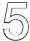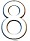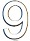# User ForumSubject :IMO    Class : Class 4

Select the odd one out.

ABCDHow is 8 the answer?

## Ans 1:

Class : Class 6
it is D and C as 8 is Symmetrical and others are not and 2 3=5, 5 3 = 8, 8 3=11 but the last option is 9 and 8 1=9

## Ans 2: (Master Answer)

Class : Class 1
Here, the figure in option 'C' is only symmetrical while the rest of the figures are not symmetrical. Hence, figure in option 'C' is the odd one out of all the four figures.

Class : Class 4

## Ans 4:

Class : Class 4
Only 8 has a line of symmetry

## Ans 5:

Class : Class 6
all the gaps are =plus signs

## Ans 6:

Class : Class 4
It would be (5) because it is only a prime number.

Class : Class 4
YEP

## Ans 8:

Class : Class 4
Here 2 3=5 and if we continue like this then 5 3=8 and 8 3=11 so, 9 is the odd one out and "D" is the correct answer.

## Ans 9:

Class : Class 4
Can you show where is symmetrical line in "D" that means 9